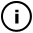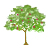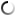# Friday, September 11, 2009

## The well

// just type here
// and click "submit" when done

void setup() {
size(600,600);
smooth();
fill(255,0,0,70);
noStroke();
}

void draw() {
//background(255,255,255,10);
// Draw points
for( int i = 0; i < 100; i++ ) {
float distance = 300.0 - abs(randomNormal()) * 50;
float angle = random(0,2*PI);
int x = int(distance * cos(angle));
int y = int(distance * sin(angle));
ellipse(width/2+x,height/2+y,4,4);
}

}

// Implements the Marsaglia Polar Method, as described in wikipedia, but only returns one of the values.
float randomNormal()
{
float x = 1.0, y = 1.0,
s = 2.0; // s = x^2 + y^2
while(s >= 1.0)
{
x = random(-1.0f, 1.0f);
y = random(-1.0f, 1.0f);
s = x*x + y*y;
}
return x * sqrt(-2.0f * log(s)/s);
}

## infosubmitted by: DARYL_Gamma
views:

This one is kind of useful cause includes a normal gaussian number generator that I found on the processing forum.This sketch has a parent
Tags: random, normal, gaussian

## commentsloading...